This is a note of the first course of the “Deep Learning Specialization” at Coursera. The course is taught by Andrew Ng.

Almost all materials in this note come from courses’ videos. The note combines knowledge from course and some of my understanding of these konwledge. I’ve reorganized the structure of the whole course according to my understanding. Thus, it doesn’t strictly follow the order of videos.

In this note, I will keep all functions and equations vectorized (without for loop) as far as possible.

If you want to read the notes which strictly follows the course, here are some recommendations:

Some parts of this note are inspired from Tess Ferrandez.

Brief Intro to Deep Learning

To begin with, let’s focus on some basic concepts to gain some intuition of deep learning.

Stuctures of Deep Learning

We start with supervised learning. Here are several types of neural network (NN) in the folloing chart:

INPUT: X OUTPUT: y NN TYPE
Home features Price Standard NN
Ad, user info Click or not Standard NN
Image Objects Convolutional NN (CNN)
Audio Text Transcription Recurrent NN (RNN)
English Chineses Recurrent NN (RNN)
Image, Radar info Position of other cars Custom NN

Here are some pictures of Standard NN, Convolutional NN, Recurrent NN: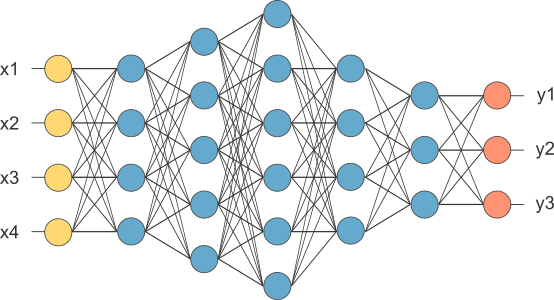Standard NNConvolutional NN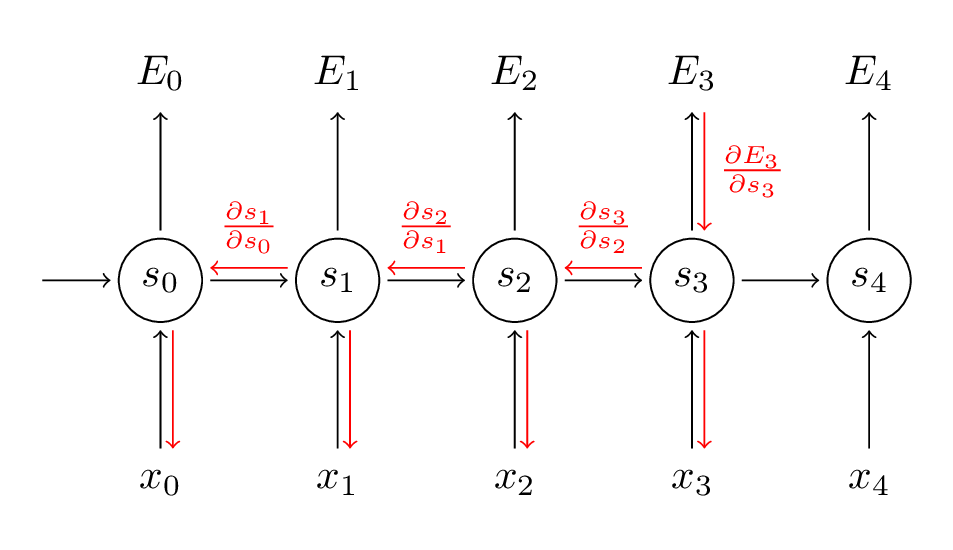Recurrent NN

Data

Neural Nework can deal with both stuctured data and unstructured data. The following will give you an intuition of both kinds of data.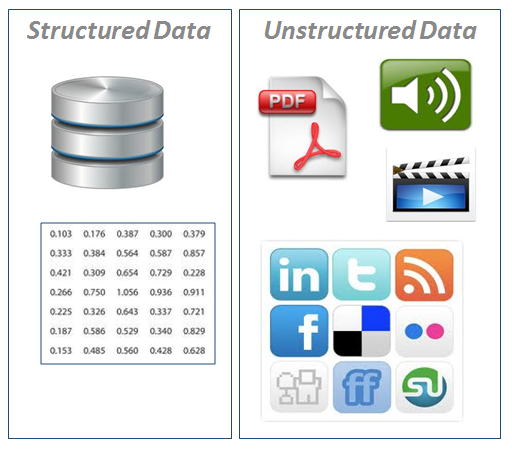Structured and Unstructured Data

Here are some conclusions of why deep learning is advanced comparing to traditional machine learning.

Firstly, deep learning models performs better when dealing with big data. Here is a comparation of deep learning models and classic machine learing models: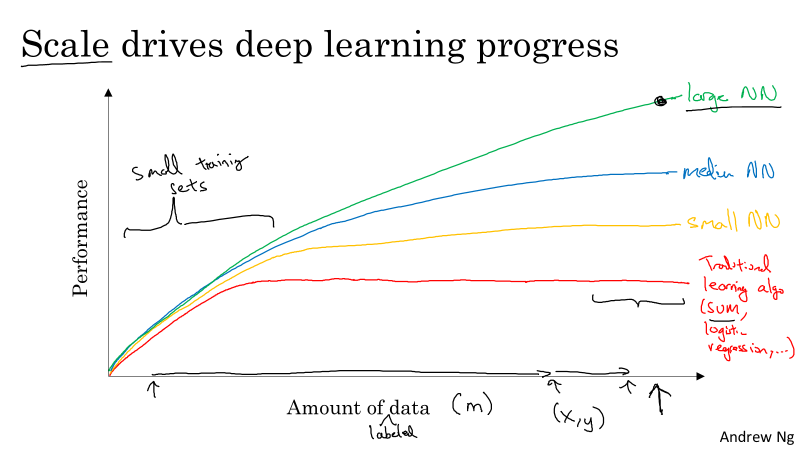Comparation between deep learning and machine learning

Secondly, thanks to the booming development of hardware and advanced algorithm, computing is much faster than before. Thus, we can implement our idea and know whether it works or not in short time. As a result, we can run the following circle much faster than we image.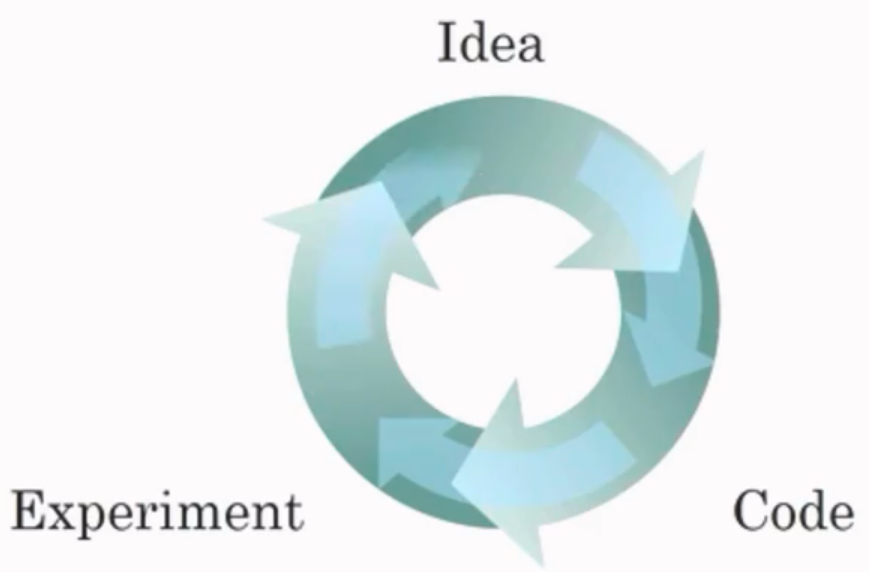Iteration process

Basic Symbols of the Course

These basic symbols will be used through out the whole specialization.Standard notations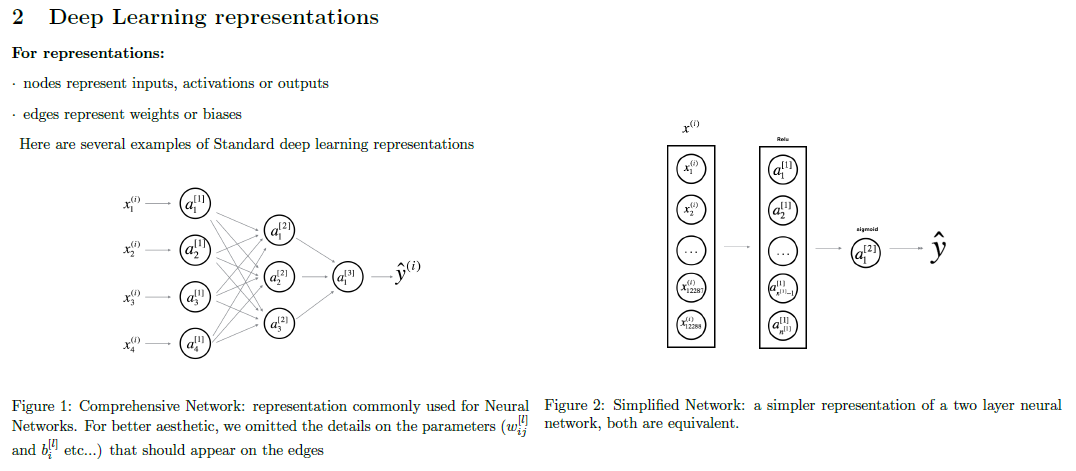Standard representation

Moreover, in this course, each input x will be stacked into columns and form the input matrix X.Input XOutput y

Neural Network

Reviewing the whole course, there are several common concepts between logistic regression and neural network (including both shallow and deep neural network). Thus, I draw conclusions on each concept and then apply them to both logistic regression and neural network.

Logistic Regression and Neural Network

First of all, here are pictures of logistic regression and neural network.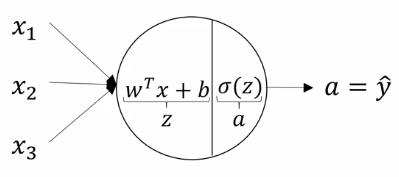Logistic Regression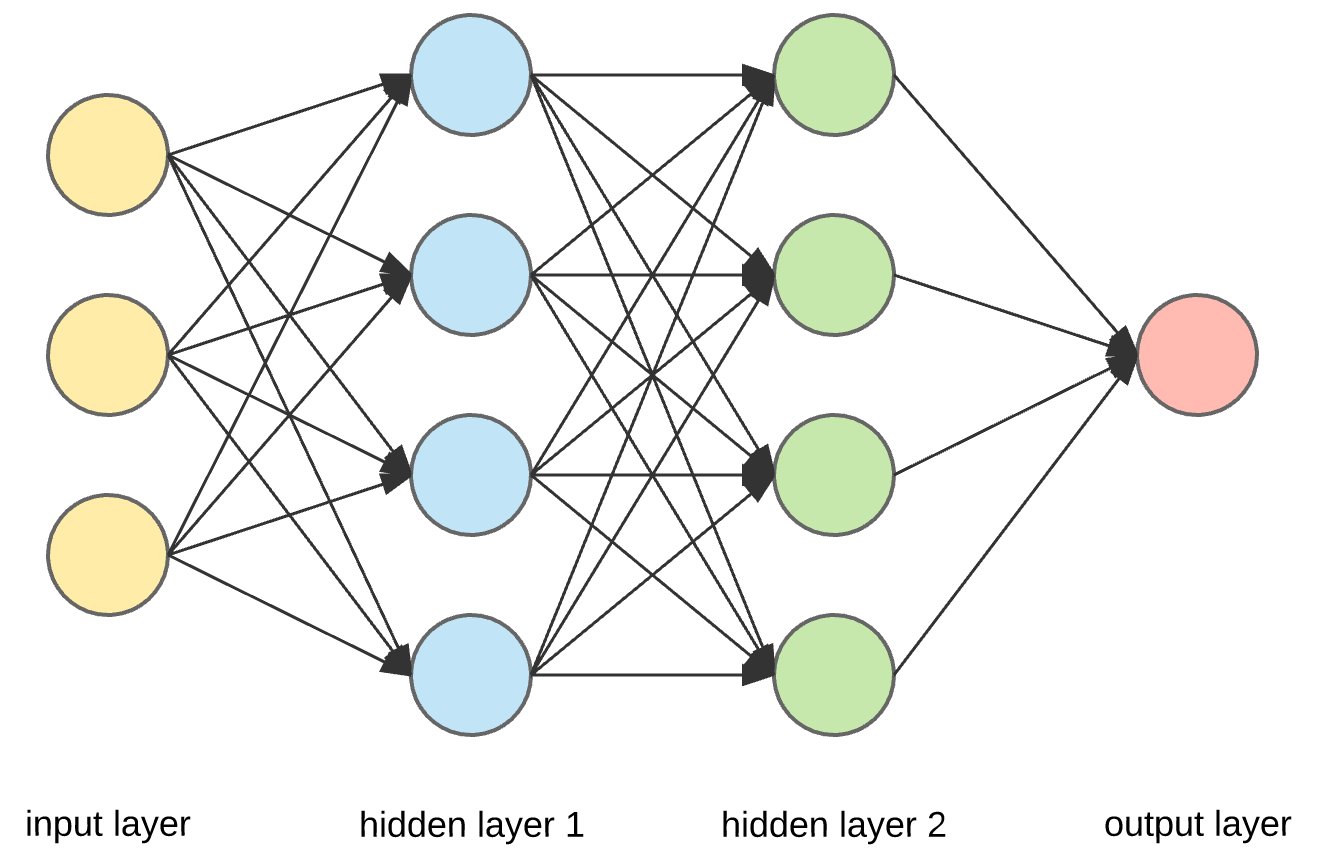Neural Network

As we can see, logistic regression is also a kind of neural network, which has input layer and output layer and does not have hidden layers, so that it is also called mini neural network. In the following sections, I will write “neural network” to represent logistic regression and neural network and use pictures similar to the second one to represent neural network.

Computation Graph

Computation graph is one of basic concepts in deep learning. By analyzing it, we could understand the whole process of computation process of neural network. The following is the basic computation graph: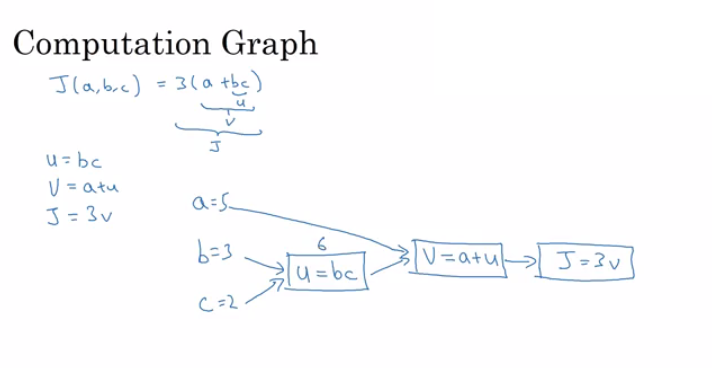In this picture, we can easily understand how $J(a,b,c)=3(a+bc)$ is computed. This process is similar to “Forward Propagation” process which I will say in next section. Moreover, in neural network, $J$ is called cost function. After computing cost function $J$, we need to feed it back to all of our parameters, such as $a$, $b$, $c$ in the picture. This process is called computing derivatives.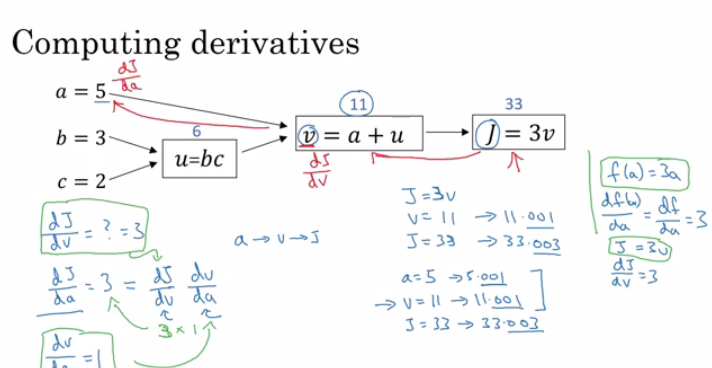By analyzing the comutation graph, we can easily compute all deviatives. According to chain rule, we can compute $\frac{dJ}{da}$ by $\frac{dJ}{dv}\frac{dv}{da}$. So do parameter $b$ and $c$. The whole derivation process is similar to “backward propagation” process in neural network.

Forward Propagation

Computation on single neuronComputation on single neuron

For every single neuron, the computing process is the same as the logistic regression. Logistic regression is basically the combination of linear regression and logistic function such as sigmoid. It has one input layer, x, and one output layer, a or $\hat{y}$.

The linear regression equation is: $z = w^Tx+b$
The sigmoid function equation is: $a = \sigma( z )$
The combination euquation is:   $\hat{y} = a = \sigma( w^Tx + b )$

The whole process on Neural Network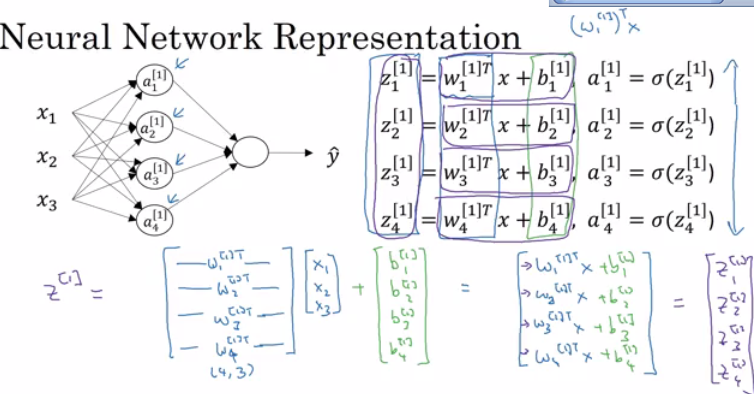Forward Propagation

This is an example of neural network. Since it only has one hidden layer, it’s also called shallow neural network. The forward propagation process means that we compute the graph from left to the right in this picture.

The whole process when computing the 1st layer (hidden layer) is as the following:

\begin{align}
Z^{} & = W^{T}X + b^{} \\
A^{} & = \sigma( Z^{} )
\end{align}

In these equations:

• $W^{T}$ is a $4 \times 3$ matrix. It is also written as $W^{}$. Its shape is always $n^{[l]} \times n^{[l - 1]}$.
• $X$ is a $3 \times m$ matrix. Sometimes it is also called $A^{}$.
• $b^{}$ is a $4 \times m$ matrix. Its shape is always $n^{[l]} \times m$.
• $A^{}$ is a $4 \times m$ matrix. Its shape is always $n^{[l]} \times m$.
• $\sigma$ is an element-wise function. It is called activation function.

For each layer, it just repeats what previous layers do until the last layer (output layer).

Cost function

Here is a definition of loss function and cost function.

• Loss function computes a single training example.
• Cost function is the average of the loss function of the whole training set.

In traditional machine learning, we use square root error as loss function, which is $L = \frac{1}{2}( \hat{y} - y )^2$. But in this case, we don’t use it since most problems we try to solve are not convex.

Here is the loss function we use:

$$L( \hat{y}, y ) = -( y \cdot log(\hat{y}) + ( 1 - y ) \cdot log( 1 - \hat{y} ) )$$

For this loss function:

• if y = 1, then $L = -y \cdot log(\hat{y})$ and it will close to 0 when $\hat{y}$ near 1.
• if y = 0, then $L = -( 1 - y ) \cdot log( 1 - \hat{y} )$ and it will close to 0 when $\hat{y}$ near 0.

Then the cost function is: $$J( w, b ) = \frac{1}{m}\sum_{i=1}^{m} L( \hat{y}, y )$$

Backward Propagation

Here, we use gradient descent as our backward propagation method.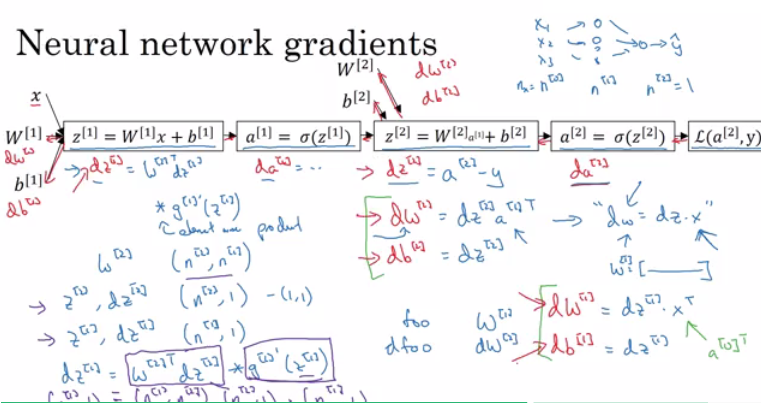Backward Propagation

As we can see in the picture, it is a simplified computation graph. The neural network is on the right-top, which is almost the same as the neural network we discussing in previous section. Backward Propagation is computing derivatives from the right to the left. By following the backward process, we can get derivatives for all parameters, including $W^{}$, $b^{}$, $W^{}$, $b^{}$.

Here I give a rough derivation example of computing gradients of parameter $W^{}$.

\begin{align}
\frac{\partial L}{\partial Z^{}} & = W^{T}\frac{\partial L}{\partial Z^{}} \cdot {\sigma}^{\prime}(Z^{}) \\
\frac{dL}{dW^{}} & = \frac{\partial L}{\partial Z^{}}\frac{dZ^{}}{dW^{}} \\
& = \frac{\partial L}{\partial Z^{}}A^{T} \\
\frac{dL}{db^{}} & = \frac{\partial L}{\partial Z^{}}\frac{dZ^{}}{db^{}} \\
& = \frac{\partial L}{\partial Z^{}}
\end{align}

It is similar to compute parameters in other layers. In these equations:

• $\frac{dL}{dW^{}}$ has the same shape as $W^{}$. So do other layers.
• $\frac{dL}{db^{}}$ has the same shape as $b^{}$. So do other layers.
• the $\cdot$ in first line is an element-wise product.

Update parameters

After computing gradients, we can update our parameters quickly.

For every parameters (Take layer1 as an example):

\begin{align}
W^{} & = W^{} - \alpha \frac{dL}{dW^{}} \\
b^{} & = b^{} - \alpha \frac{dL}{db^{}}
\end{align}

In above equations, $\alpha$ is called learning rate, which we need to determine before training.

Activation Functions

In previous sections, notation $\sigma$ is used to represent activation function. In neural network, there are five common activation functions: Sigmoid, Tanh, ReLU, Leaky ReLU, and Exponential LU.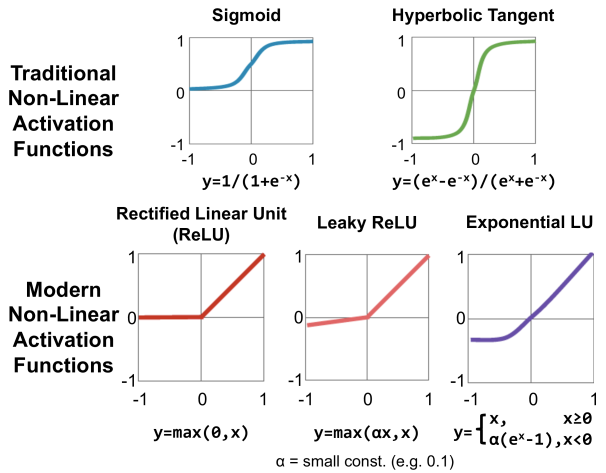Activation Functions

In the course, Prof. Andrew Ng introduces the first four activation functions.

Here are some experience on choosing those activation functions:

• Sigmoid: It is usually used in output layer to generate results between 0 and 1 when doing binary classification. In other case, you should not use it.
• Tanh: It always works better than sigmoid function since its value is between -1 and 1, so that neural network can learn more information by using it than using sigmoid function.
• ReLU: The most commonly used activation function is ReLU function. If you don’t want to use it, you can choose other ReLU derivatives, such as Leaky ReLU.